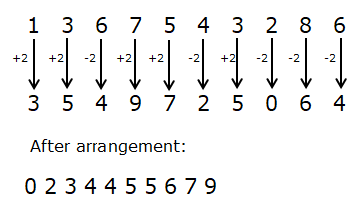# RRB Clerk Prelims Reasoning (Day-29)

## Reasoning Materials for RRB Clerk Prelims:

Dear Aspirants, Our IBPS Guide team is providing new series of Reasoning Questions for RRB Clerk Prelims 2020 so the aspirants can practice it on a daily basis. These questions are framed by our skilled experts after understanding your needs thoroughly. Aspirants can practice these new series questions daily to familiarize with the exact exam pattern and make your preparation effective.

Start Quiz

Number series

Direction (1-5): Following questions are based on the five three-digit numbers given below.

124   465   321   576   865

1) If all the digits in each of the numbers are arranged in ascending order within the number, then which of the following number is the highest number?

A) 321

B) 124

C) 576

D)  865

E) 465

2) If all the numbers are arranged in descending order from left to right, then which of the following will be the product of the 3rd digit of 2nd number from the left end and 3rd digit of 2nd number from the right end?

A) 12

B) 6

C) 10

D) 15

E) 7

3) What will be the resultant when 3rd digit of the highest number is multiplied with the 2nd digit of the lowest number?

A) 16

B) 10

C) 8

D) 24

E) 20

4) If the position of the second and the third digits of each of the numbers are interchanged, then how many odd numbers will be formed?

A) 0

B) 4

C) 3

D) 1

E) 2

5) If one is subtracted from each of the numbers, then how many numbers thus formed will be divisible by 4?

A) 4

B) 3

C) 1

D) 2

E) 0

6) In a row of 50 students facing south direction, N is 18th from the left end and O is 17th from the right end. Then how many students are between N and O?

A) 15

B) 16

C) 17

D) 14

E) 13

7) Five people A, B, C, D, and E are in different heights. A is taller than only D and C is taller than E, B is not the tallest person. Who among the following person is the 3rd tallest person?

A) A

B) C

C) B

D) E

E) Either B or E

8) If the position of the letters in the word “CORPORATION” are rearranged in such a way that the position of N remains the same position, then the first and the tenth letter are interchanged, similarly the position of the second and ninth letters are interchanged and so on, from the left end then which of the following will be the sixth from the left end after the rearrangement?

A) R

B) C

C) O

D) P

E) A

9) If the letter of the word “Employee” is arranged in alphabetical order from left to right, then which letter would be third from the right end?

A) O

B) M

C) L

D) P

E) E

10) If in a number ‘1367543286’ two is added in each odd digit and two is subtracted from each even digit after that arrange it in ascending order from left to right. After the operation which of the following digit will be fifth from the right end?

A) 6

B) 5

C) 4

D) 3

E) None of these

Directions (1-5) :

The new arrangement is- 124 456 123 567 568

After the arrangement, 2ndnumber from the left end is – 576

And 2ndnumber from the right end is – 321

So, 6*1=6

3rd digit of the highest number is – 5

2nd digit of the lowest number is – 2

Their product is 10

After the arrangement, the series is – 142 456 312 567 856

After the arrangement, the series is – 123 464 320 575 864

Directions (6-7):

50-(18+17) = 15

A is taller than only D, so we assume that D is the shortest person and A is 2nd shortest person. (A > D)C is taller than E, (C > E) B is not the tallest person. From these condition, we can find, C > E, B > A > D.

Directions (8-10) :

CORPORATION

OITAROPROCN

Employee -> eeelmopy